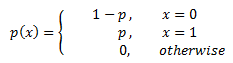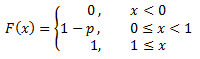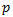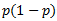AnyLogic

# bernoulli

 Probability mass functionDistributionMeanVarianceThe Bernoulli distribution is a discrete probability distribution with two possible outcomes ("success" and "failure"). It takes value 1 with success probability p and value 0 with failure probability q = 1 − p.

### Example

bernoulli(0.6) results in 60% chance of success (returns 1 with 60% probability).

### bernoulli(double p)

Description
Generates a sample of the Bernoulli distribution, i.e. 1 with probability p and 0 with probability 1 - p.
Parameters
Name Type of value Description
p double The probability of 1.
Result
Type Description
int The generated sample.

### bernoulli(double p, java.util.Random r)

Description
Generates a sample of the Bernoulli distribution using the specified random number generator.
Parameters
Name Type of value Description
p double The probability of 1.
r java.util.Random The random number generator.
Result
Type Description
int The generated sample.